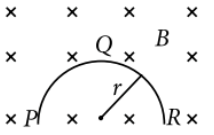Motional EMF
Question

# A thin semicircular conducting ring (PQR) of radius r is falling with its plane vertical in a horizontal magnetic field B, as shown in the figure.The potential difference developed across the ring when its speed $\nu$, is

Easy
Solution

## Motional emf induced in the semicircular ring PQR is equivalent to the motional emf induced in the imaginary conductor PR.                                    (l= PR = 2r)Therefore, potential difference developed across the ring is 2rBv with R is at highe rpotential.

Get Instant Solutions